Function Repository Resource:

# FlipFunction

Reverse the order of two arguments of a function

Contributed by: Nikolay Murzin
 ResourceFunction["FlipFunction"][f] gives a version of f in which its first two arguments are exchanged. ResourceFunction["FlipFunction"][f,{i,j}] exchanges arguments at positions i and j.

## Details and Options

This function is closely related to the C combinator of the B, C, K, W combinatory logic system discovered by Haskell Curry in 1930.

## Examples

### Basic Examples (3)

Make a flipped Power function:

 In:=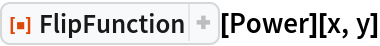Out=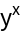Flip the order in which StringJoin joins its string arguments:

 In:=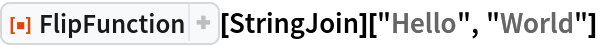Out=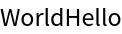Flip the first and last arguments:

 In:=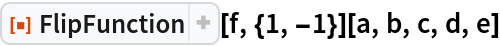Out=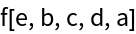### Properties and Relations (1)

For two arguments, FlipFunction[f] is equivalent to Curry[f]:

 In:=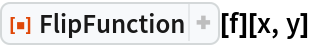Out=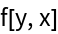In:=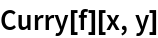Out=N. Murzin

## Version History

• 1.0.0 – 02 March 2020